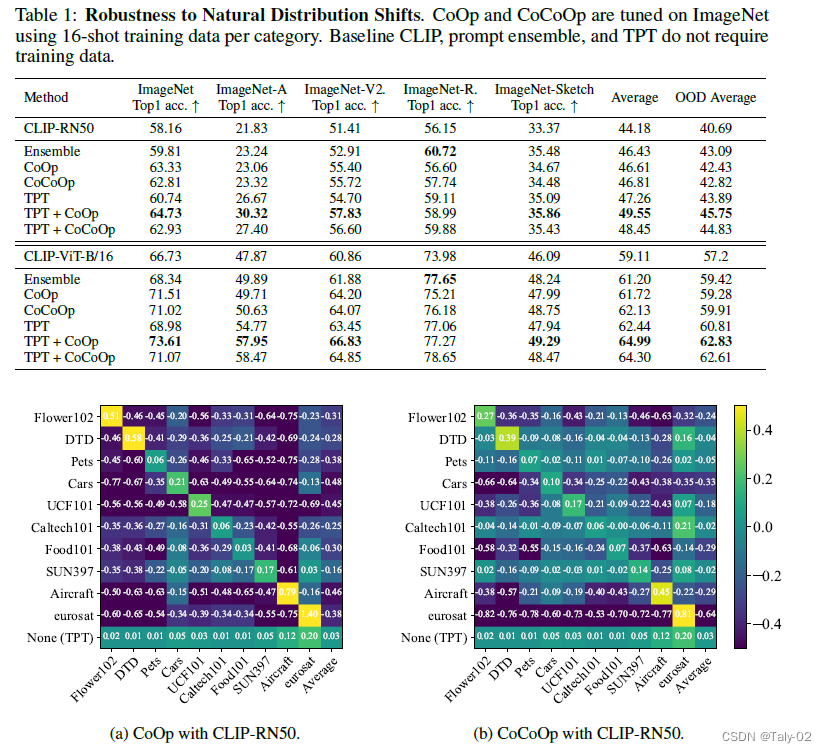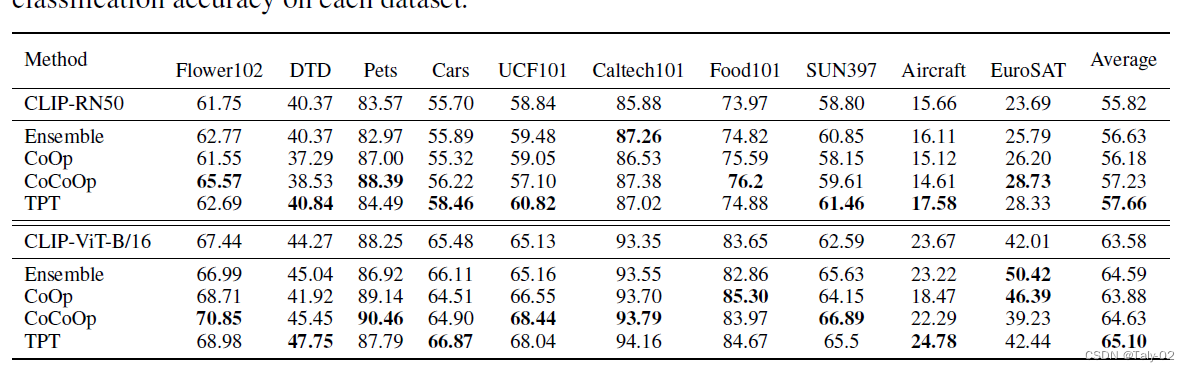# NeurIPS 2022： Test-Time Prompt Tuning for Zero-Shot Generalization in Vision-Language Models¶

## 2. 引言¶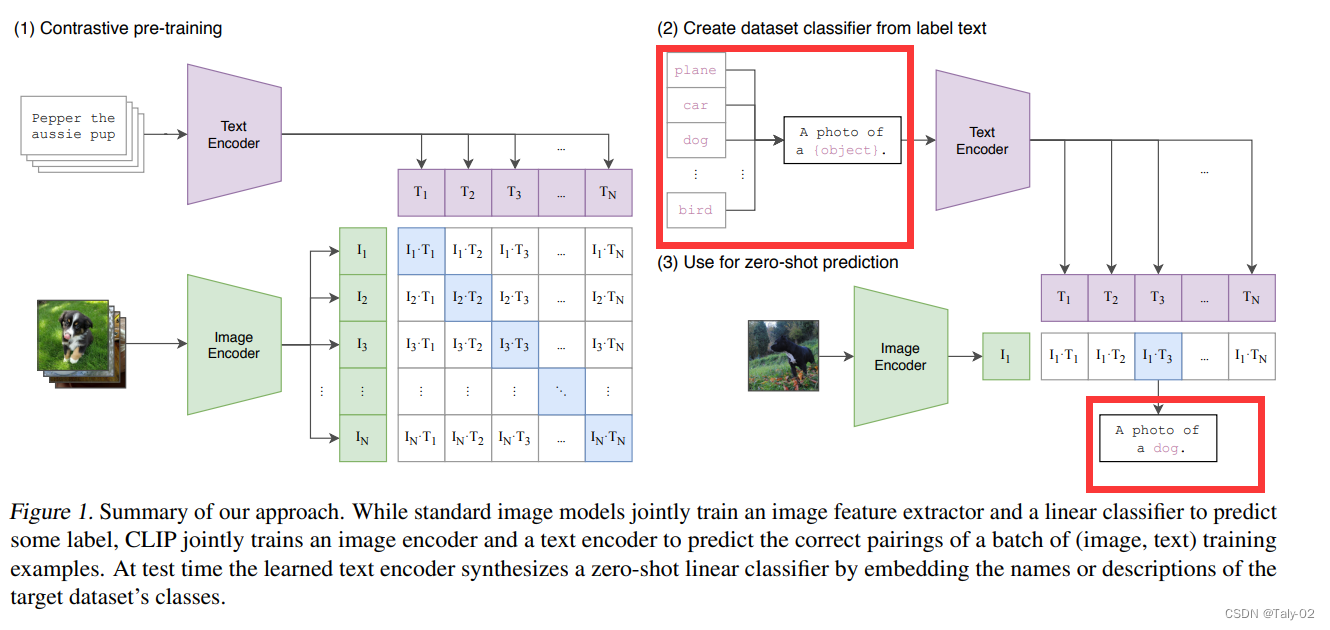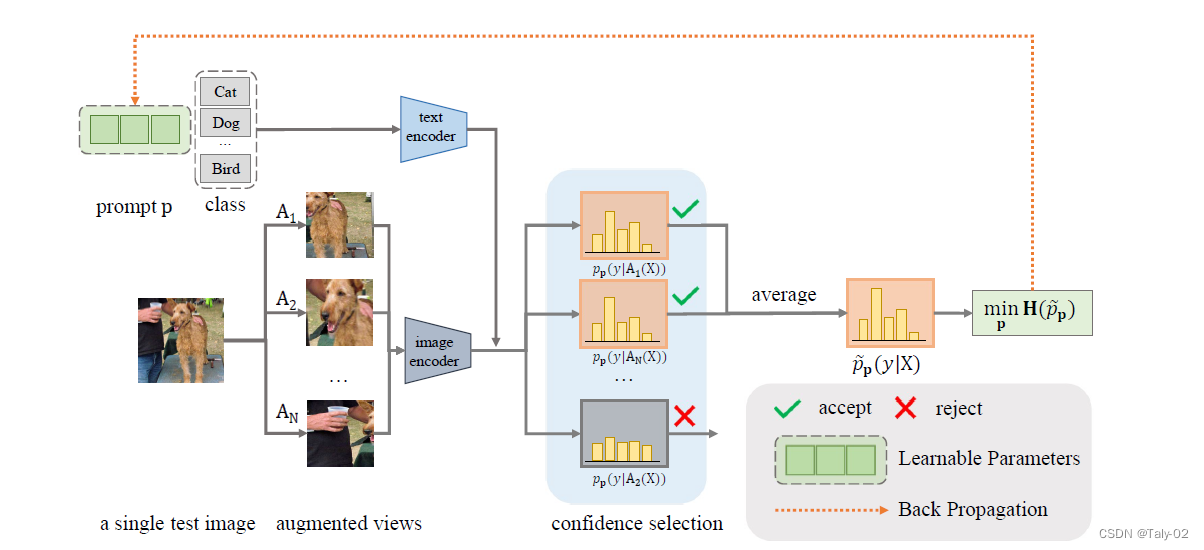## 3. 方法¶

p^*=\arg \min _p \mathcal{L}\left(\mathcal{F}, \boldsymbol{p}, X_{\text {test }}\right)

p^*=\arg \min _{\boldsymbol{p}}-\sum_{i=1}^K \tilde{p}_{\boldsymbol{p}}\left(y_i \mid X_{\text {test }}\right) \log \tilde{p}_{\boldsymbol{p}}\left(y_i \mid X_{\text {test }}\right), \\ \text { where } \tilde{p}_{\boldsymbol{p}}\left(y_i \mid X_{\text {test }}\right)=\frac{1}{N} \sum_{i=1}^N p_{\boldsymbol{p}}\left(y_i \mid \mathcal{A}_i\left(X_{\text {test }}\right)\right) \text {. }

\tilde{p}_{\boldsymbol{p}\left(y \mid X_{\text {test }}\right)}=\frac{1}{\rho N} \sum_{i=1}^N \mathbb{1}\left[\mathbf{H}\left(p_i\right) \leq \tau\right] p_{\boldsymbol{p}}\left(y \mid \mathcal{A}_i\left(X_{\text {test }}\right)\right)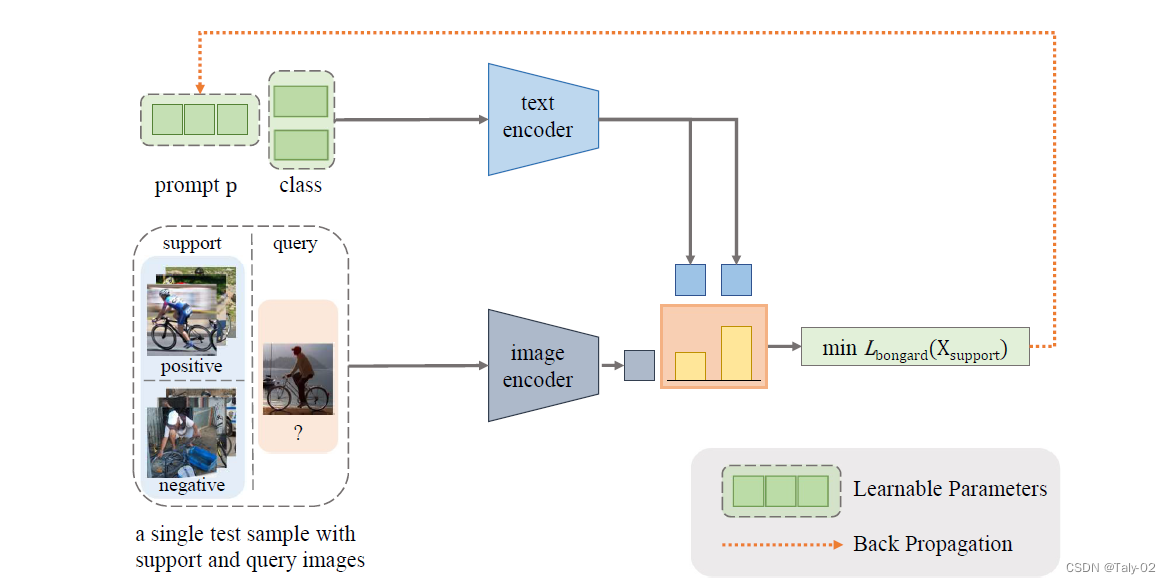## 4. 实验¶